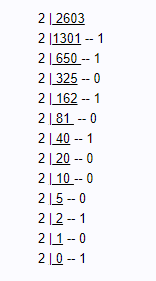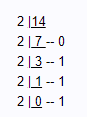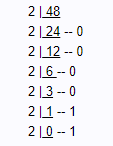Convert hexadecimal to binary is a conversion of a number in a hexadecimal number system to an equivalent number in the binary number system. The binary number system is a widely used number system. Its main application is its usage in computers. Computers can understand only binary language. So all other number systems given by the user will be stored in binary form in computers. Thus, the conversion of hexadecimal to binary is very important.

Here it is not possible to convert it directly, we will convert hexadecimal to decimal then that decimal number is converted to binary. Before going into the conversion let us discuss binary and hexadecimal numbers.

Binary Numbers: The number which uses only the digits 0 and 1 and data in this system will be the combination of 0’s and 1’s. It uses only 2 digits so it is called binary numbers. It is denoted by b2, where b is any binary number.

Examples: 1) 0101112 2 ) 001110112 3) 1112

Hexadecimal Number: Hexa means 16. In the hexadecimal number system, it uses 16 digits. It consists of numbers and alphabets. It includes numbers 0, 1, 2, 3, 4, 5, 6, 7, 8, 9 and A, B, C, D, E, F; total 16 digits. It is denoted by s16, where s is a hexadecimal number.

Examples: 1) A116 2) EE916 3) FD65416

## Hex to Binary Table

Here is the table for hex to binary conversion. The table is given for the first few numbers up to 16.

 Hexadecimal Number Binary Number 0 0 1 1 2 10 3 11 4 100 5 101 6 110 7 111 8 1000 9 1001 A 1010 B 1011 C 1100 D 1101 E 1110 F 1111

## How to Convert Hexadecimal to Binary Number?

To convert a hexadecimal number into its equivalent binary number, follow the steps given here:

• Step 1: Take given hexadecimal number
• Step 2: Find the number of digits in the decimal
• Step 3: If it has n digits, multiply each digit with 16n-1 where the digit is in the nth position
• Step 4: Add the terms after multiplication
• Step 5: The result is the decimal number equivalent to the given hexadecimal number. Now we have to convert this decimal to binary number.
• Step 6: Divide the decimal number with 2
• Step 7: Note the remainder
• Step 8: Do the above 2 steps for the quotient till the quotient is zero
• Step 9: Write the remainders in the reverse order.
• Step 10: The result is the required binary number.

Hence, from the above steps it is clear that how to convert any hexadecimal number into binary, i.e. first, we need to convert hexadecimal to decimal number and then decimal to binary.

## Hexa To Binary Examples

Question 1: Convert A2B16 to an equivalent binary number.

Solution: Given hexadecimal number = A2B16

First, convert the given hexadecimal to the equivalent decimal number.

A2B16 = (A × 162) + (2 × 161) + (B × 160)

= (A × 256) + (2 × 16) + (B × 1)

= (10 ×256) + 32 + 11

= 2560 + 43

= 2603(Decimal number)

Now we have to convert 260310 to binaryThe binary number obtained is 1010001010112

Hence, A2B16 = 1010001010112

Question 2: Convert E16 to an equivalent binary number.

Solution: Given, a hexadecimal number is E.

First, convert the given hexadecimal to the equivalent decimal number.

E16 = E × 160

= E × 1

= E

=14 (Decimal number)

Now we have to convert 1410 to binary number.The binary number obtained is 11102

Hence, E16 = 11102

Question 3: Convert 3016 to an equivalent binary number.

Solution: Given the hexadecimal number is 30

First, convert the given hexadecimal to the equivalent decimal number.

3016 = (3 × 161) + (0 × 160)

= 48 + 0

= 48(Decimal number)

Now we have to convert 4810 to binary.

Therefore,The binary number is 1100002

Hence, 3016 = 1100002

### Practice Problems

• Convert FDA16 to an equivalent binary number
• Convert 98B16 to an equivalent binary number
• Convert 5C16 to an equivalent binary number
• Convert 10016 to an equivalent binary number